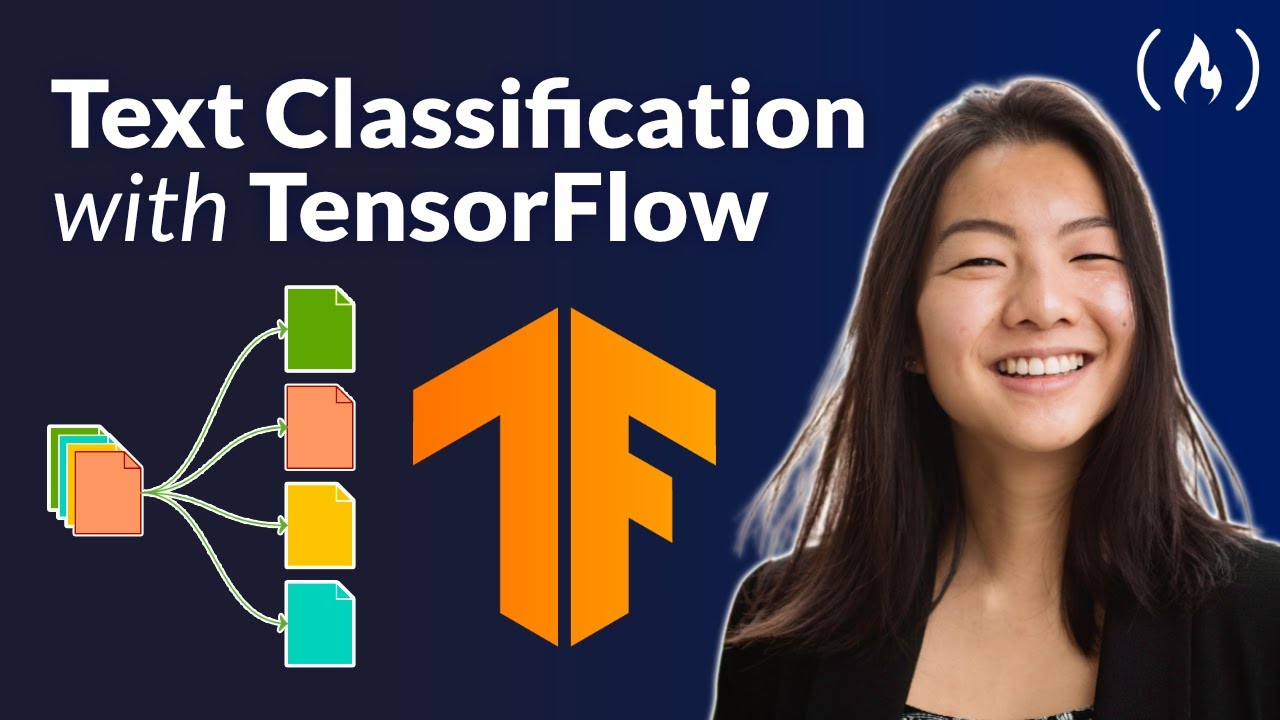# Python TensorFlow for Machine Learning – Neural Network Text Classification TutorialThis course will give you an introduction to machine learning concepts and neural network implementation using Python and TensorFlow.

This course will give you an introduction to machine learning concepts and neural network implementation using Python and TensorFlow. Kylie Ying explains basic concepts, such as classification, regression, training/validation/test datasets, loss functions, neural networks, and model training. She then demonstrates how to implement a feedforward neural network to predict whether someone has diabetes, as well as two different neural net architectures to classify wine reviews.